# Overview

Population time plots can be extremely informative graphical displays of survival data. They should be the first step in your exploratory data analyses. We facilitate this task in the `casebase` package by providing a `plot` method for objects returned by the `popTime` function. This is done in two steps:

1. Pass your dataset to the `casebase::popTime` function and specify the column names corresponding to
• the time of event
• the event status
• exposure (optional)

This will create an object of class `popTime` with an exposure attribute if you specify a value for the `exposure` argument.

1. Pass the object of class `popTime` to the `plot` function

In this vignette we show how to create population-time plots for the two datasets that ship with the `casebase` package, as well as several well known survival datasets from the `survival` package. For a more detailed description of how to modify the aesthetics of a population time plot, please refer to the Customizing Population Time Plots vignette.

``````library(survival)
library(casebase)
library(ggplot2)
library(data.table)``````

# European Randomized Study of Prostate Cancer Screening Data

For our first example, we make use of the European Randomized Study of Prostate Cancer Screening (ERSPC) data which ships with the `casebase` package (see `help("ERSPC", package = "casebase")` for details about this data).

``````data("ERSPC")
``````##   ScrArm Follow.Up.Time DeadOfPrCa
## 1      1         0.0027          0
## 2      1         0.0027          0
## 3      1         0.0027          0
## 4      0         0.0027          0
## 5      0         0.0027          0
## 6      0         0.0027          0``````

We first pass this dataset to the `popTime` function. If you do not specify the `time` and `event` arguments, this function will try to guess them using regular expressions. See the Details section of `help("popTime", package = "casebase")` for how we try to guess these inputs.

``pt_object <- casebase::popTime(ERSPC, event = "DeadOfPrCa")``
``## 'Follow.Up.Time' will be used as the time variable``

We can see its contents and its class:

``head(pt_object)``
``````##    ScrArm   time event original.time original.event event status ycoord yc
## 1:      1 0.0027     0        0.0027              0     censored 159893  0
## 2:      1 0.0027     0        0.0027              0     censored 159892  0
## 3:      1 0.0027     0        0.0027              0     censored 159891  0
## 4:      0 0.0027     0        0.0027              0     censored 159890  0
## 5:      0 0.0027     0        0.0027              0     censored 159889  0
## 6:      0 0.0027     0        0.0027              0     censored 159888  0
##    n_available
## 1:           0
## 2:           0
## 3:           0
## 4:           0
## 5:           0
## 6:           0``````
``class(pt_object)``
``##  "popTime"    "data.table" "data.frame"``

The `casebase` package has a `plot` method for objects of class `popTime`:

``````# plot method for objects of class 'popTime'
plot(pt_object)``````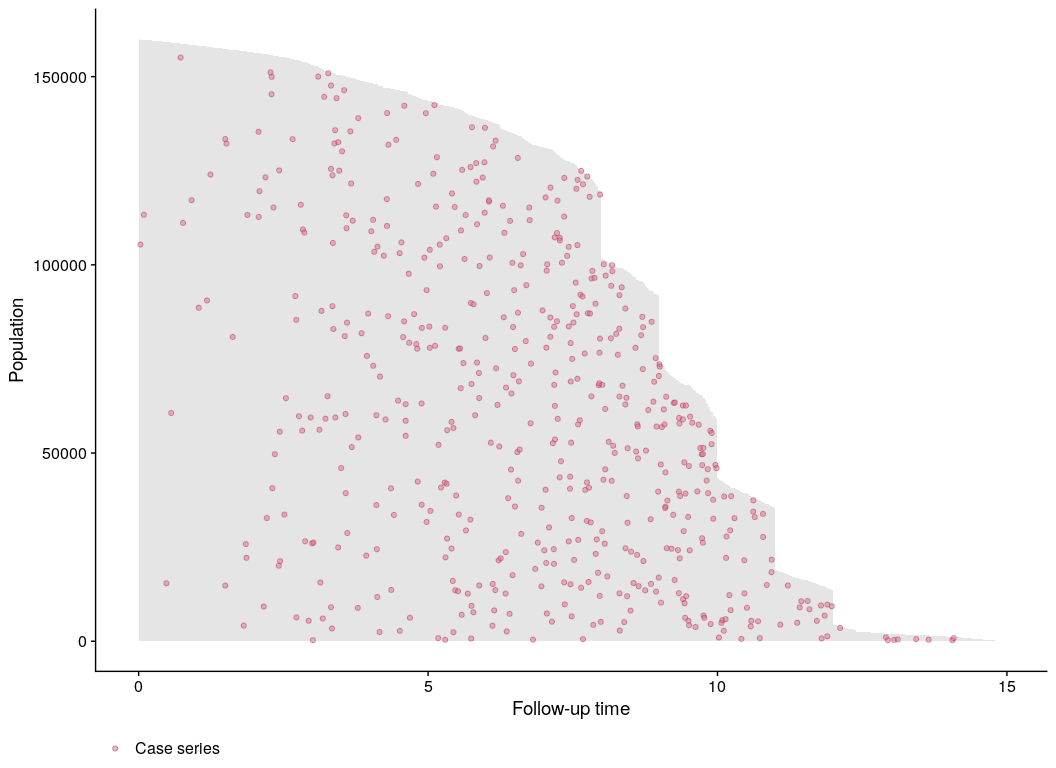One benefit from these plots is that it allows you to see the incidence density. This can be seen from the distribution of the red dots in the above plot. We can see that more events are observed later on in time. Therefore a constant hazard model would not be appropriate in this instance as it would overestimate the cumulative incidence earlier on in time, and underestimate it later on.

The unique ‘step shape’ of the population time plot is due to the randomization of the Finnish cohorts which were carried out on January 1 of each of the 4 years 1996 to 1999. This, coupled with the uniform December 31 2006 censoring date, lead to large numbers of men with exactly 11, 10, 9 or 8 years of follow-up.

It is important to note that the red points are random distributed across the gray area for each time of event. That is, imagine we draw a vertical line at a specific event time. We then plot the red point at a randomly sampled y-coordinate along this vertical line. This is done to avoid having all red points along the upper edge of the plot (because the subjects with the least amount of observation time are plotted at the top of the y-axis). By randomly distributing them, we can get a better sense of the incidence density.

## Exposure Stratified Population Time Plot

Often the observations in a study are in specific groups such as treatment arms. It may be of interest to compare the population time plots between these two groups. Here we compare the control group and the screening group. We create exposure stratified plots by specifying the `exposure` argument in the `popTime` function:

``````# Appropriately label the factor variable so that these labels appear in the
# stratified population time plot
ERSPC\$ScrArm <- factor(ERSPC\$ScrArm,
levels = c(0,1),
labels = c("Control group", "Screening group"))
pt_object_strat <- casebase::popTime(ERSPC,
exposure = "ScrArm")``````
``## 'Follow.Up.Time' will be used as the time variable``

We can see its contents, class and that it has an exposure attribute:

``head(pt_object_strat)``
``````##           ScrArm   time event original.time original.event event status ycoord
## 1: Control group 0.0027     0        0.0027              0     censored  88232
## 2: Control group 0.0027     0        0.0027              0     censored  88231
## 3: Control group 0.0027     0        0.0027              0     censored  88230
## 4: Control group 0.0027     0        0.0027              0     censored  88229
## 5: Control group 0.0137     0        0.0137              0     censored  88228
## 6: Control group 0.0137     0        0.0137              0     censored  88227
##    yc n_available
## 1:  0           0
## 2:  0           0
## 3:  0           0
## 4:  0           0
## 5:  0           0
## 6:  0           0``````
``class(pt_object_strat)``
``##  "popTime"    "data.table" "data.frame"``

We can also see that the `pt_object_strat` has an exposure attribute which contains the name of the exposure variable in the dataset:

``attr(pt_object_strat, "exposure")``
``##  "ScrArm"``

The plot method for objects of class `popTime` will use this exposure attribute to create exposure stratified population time plots:

``plot(pt_object_strat)``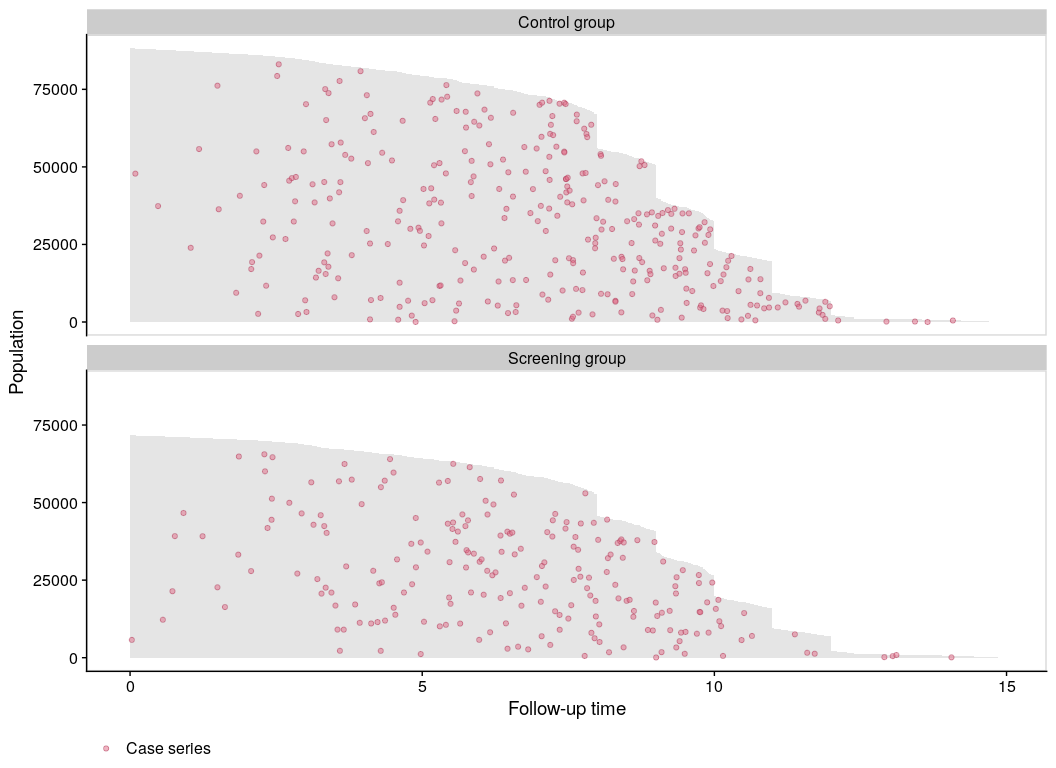We can also plot them side-by-side using the `facet.params` argument, which is a list of arguments that are passed to the `facet_wrap()` function in the `ggplot2` package:

``````plot(pt_object_strat,
facet.params = list(ncol = 2))``````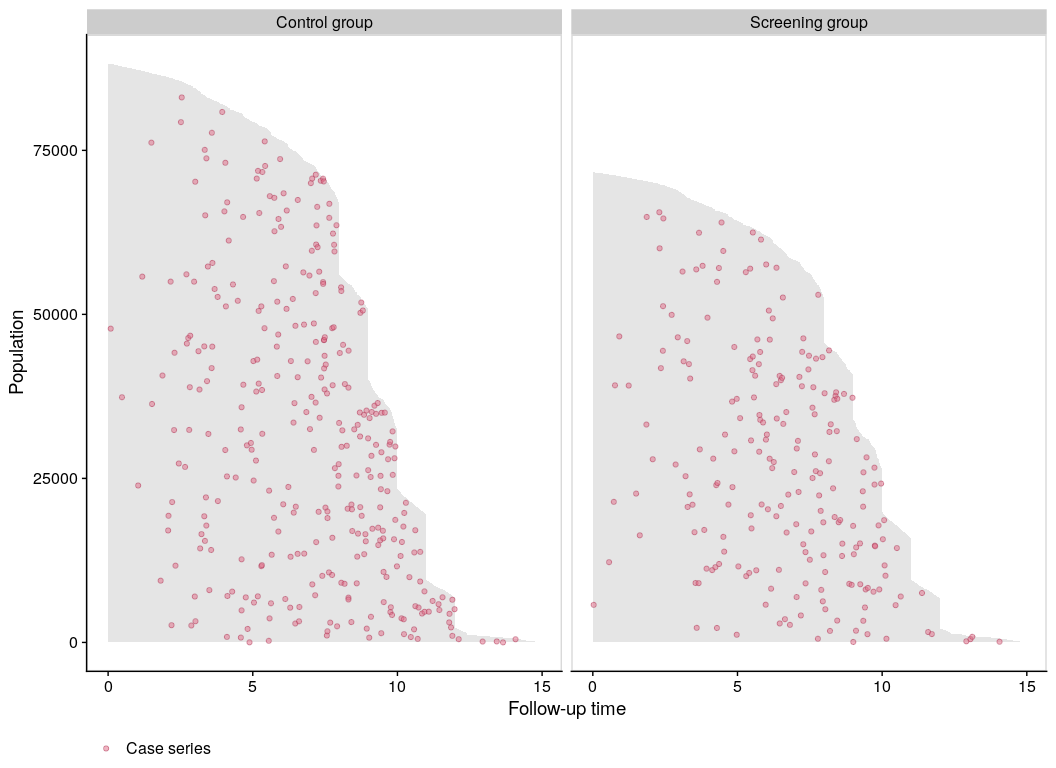## Plotting the base series

To illustrate the casebase sampling methodology, we can also plot the base series using the `add.base.series` function:

``````plot(pt_object_strat,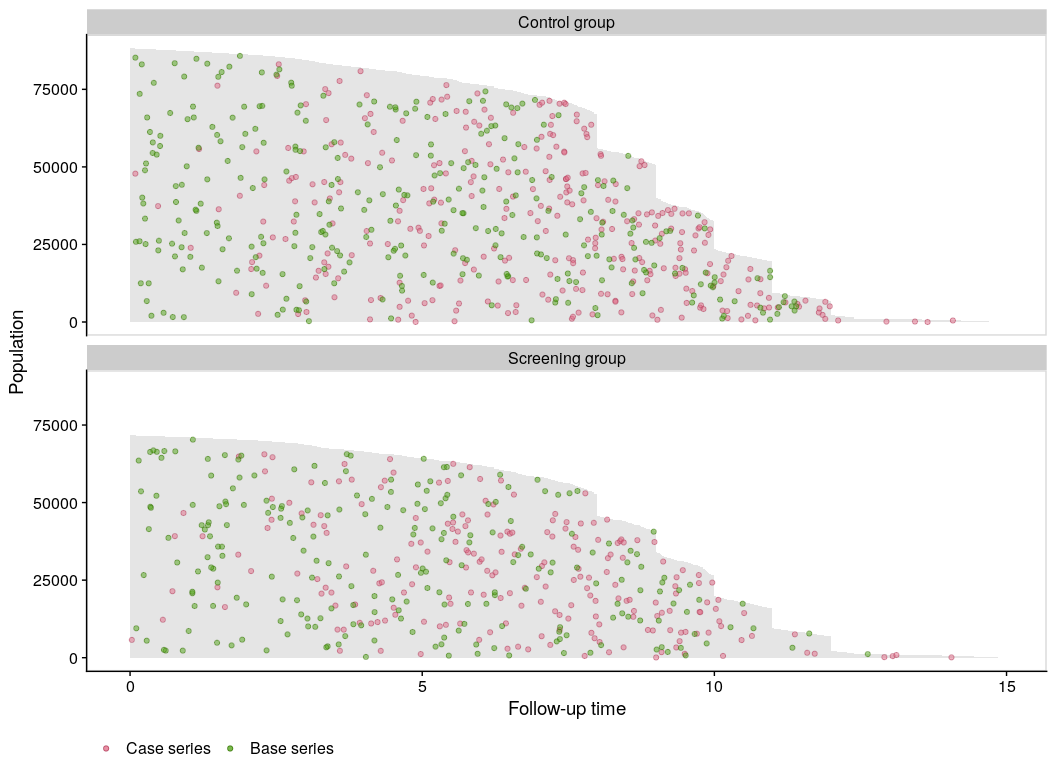Note that the `theme.params` argument is a list of arguments passed to the `ggplot2::theme()` function.

# Stem Cell Data

Next we show population time plots when there is a competing event. The `bmtcrr` dataset contains information on patients who underwent haematopoietic stem cell transplantation for acute leukaemia. This data is included in the `casebase` package. See `help("bmtcrr", package = "casebase")` for more details. We will use this dataset to further illustrate the fundamental components of a population time plot.

Note that for this dataset, the `popTime` fails to identify a `time` variable if you didn’t specify one:

``````# load data
data(bmtcrr)
str(bmtcrr)``````
``````## 'data.frame':    177 obs. of  7 variables:
##  \$ Sex   : Factor w/ 2 levels "F","M": 2 1 2 1 1 2 2 1 2 1 ...
##  \$ D     : Factor w/ 2 levels "ALL","AML": 1 2 1 1 1 1 1 1 1 1 ...
##  \$ Phase : Factor w/ 4 levels "CR1","CR2","CR3",..: 4 2 3 2 2 4 1 1 1 4 ...
##  \$ Age   : int  48 23 7 26 36 17 7 17 26 8 ...
##  \$ Status: int  2 1 0 2 2 2 0 2 0 1 ...
##  \$ Source: Factor w/ 2 levels "BM+PB","PB": 1 1 1 1 1 1 1 1 1 1 ...
##  \$ ftime : num  0.67 9.5 131.77 24.03 1.47 ...``````
``````# table of event status by exposure
table(bmtcrr\$Status, bmtcrr\$D)``````
``````##
##     ALL AML
##   0  17  29
##   1  28  28
##   2  28  47``````
``````# error because it can't determine a time variable
popTimeData <- popTime(data = bmtcrr)``````
``## Error in checkArgsTimeEvent(data = data, time = time, event = event): data does not contain time variable``

In this case, you must be explicit about what the time variable is:

``popTimeData <- popTime(data = bmtcrr, time = "ftime")``
``## 'Status' will be used as the event variable``
``class(popTimeData)``
``##  "popTime"    "data.table" "data.frame"``

## Plotting the follow-up times for each observation

We first plot that area on the graph representing the observed follow-up time. Fundamentally, this area is constructed by plotting a line for each individual, where the length of each line represents their follow-up time in the cohort. The follow-up times are plotted from top (shortest follow-up time) to bottom (longest follow-up time). In practice, we instead plot a polygon using the `ggplot2::geom_ribbon()` function. The following figure shows this area for the `bmtcrr` dataset. Note that we must specify `add.case.series = FALSE` because the default is to add the case series:

``````plot(popTimeData,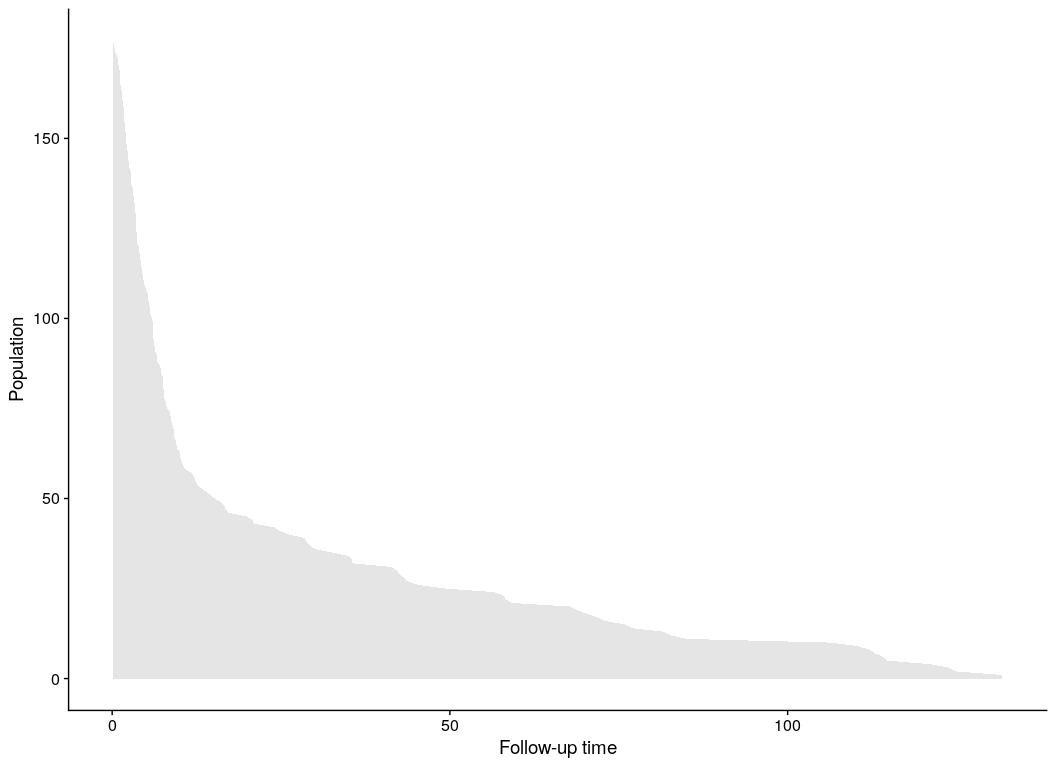Note that we can change the aesthetics of the area by using the `ribbon.params()` argument as follows. These arguments are passed to the `ggplot2::geom_ribbon()` function:

``````plot(popTimeData,
ribbon.params = list(color = "red", fill = "blue", size = 2, alpha = 0.2))``````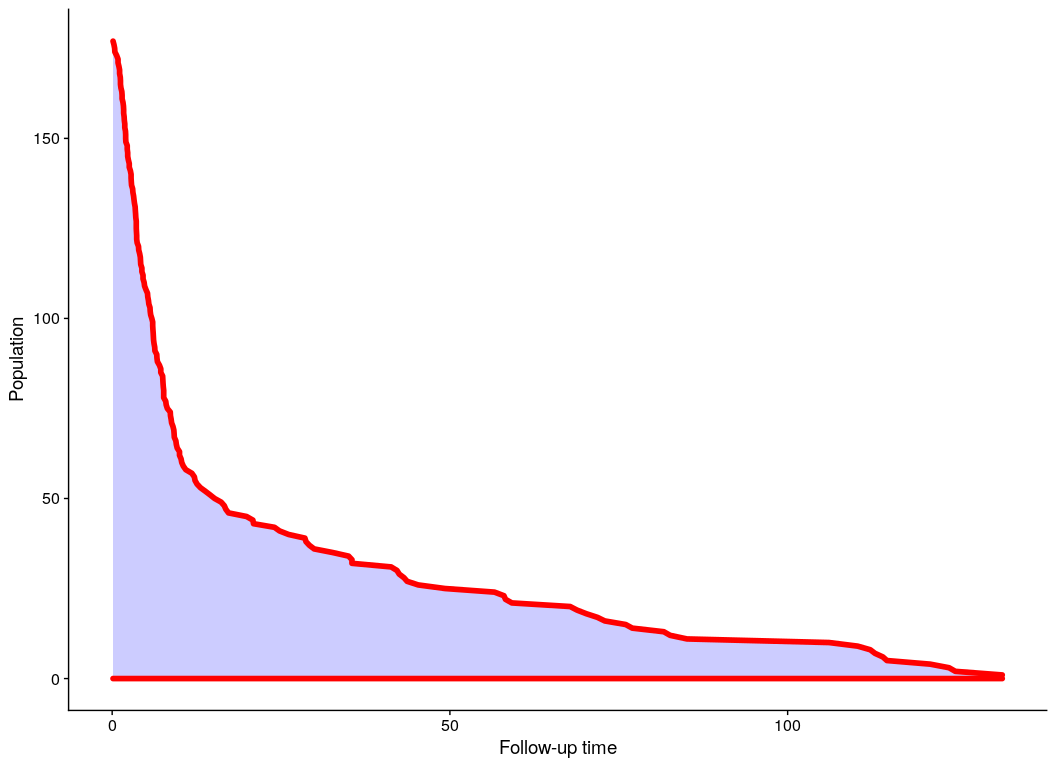## Plot the Case Series

Next we add the case series. Note that because the `Status` column has a competing event (coded as 2), we must specify `comprisk = TRUE` (even if you don’t want to plot the competing event):

``````plot(popTimeData,
comprisk = TRUE)``````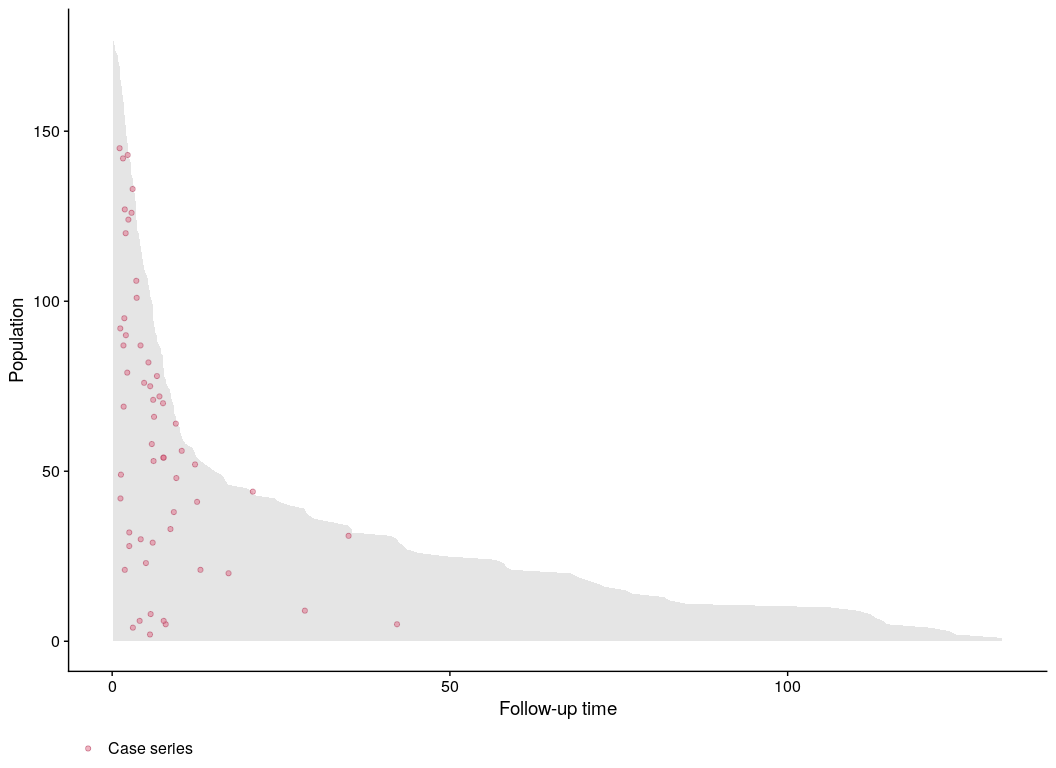In the above plot we can clearly see many of the deaths occur at the beginning, so in this case, a constant hazard assumption isn’t valid. This information is useful when deciding on the type of model to use.

## Plot the Base Series

We can now add the base series with the `add.base.series` argument. Internally, the `plot` method calls the `casebase::sampleCaseBase` function to sample the base series from the total person moments. This requires us to specify the ratio of base series to case series in the `ratio` argument which we will leave at its default of 1. A legend is also added by default:

``````plot(popTimeData,
comprisk = TRUE)``````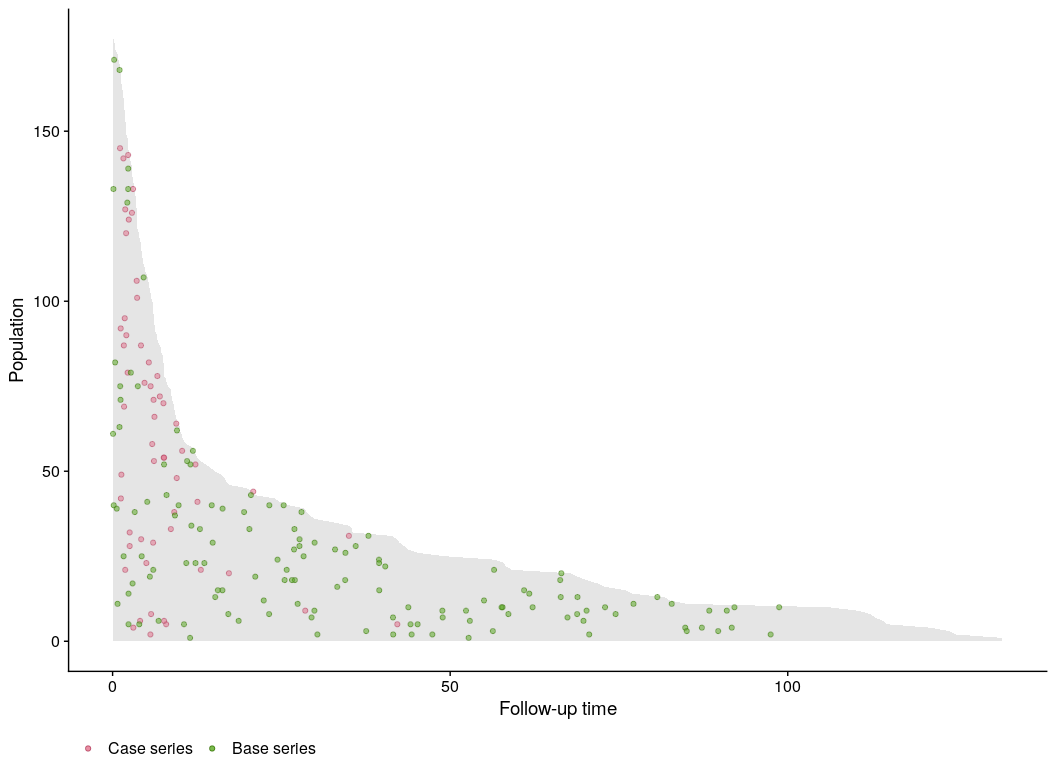## Plot the Competing event

We specify the `add.competing.event = TRUE` in order to also plot the competing event. Note, that like the case series, the competing event is sampled randomly on the vertical axis in order to see the incidence density.

``````plot(popTimeData,
comprisk = TRUE)``````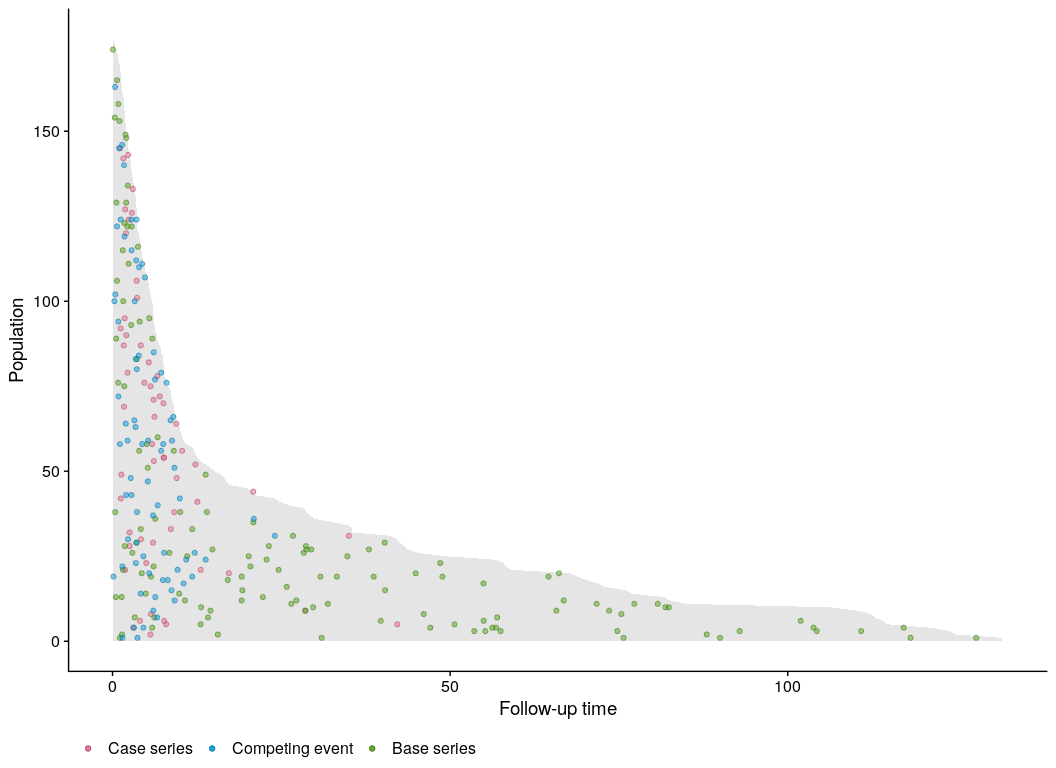We can also only plot the case series and competing event (or any combination):

``````plot(popTimeData,
comprisk = TRUE)``````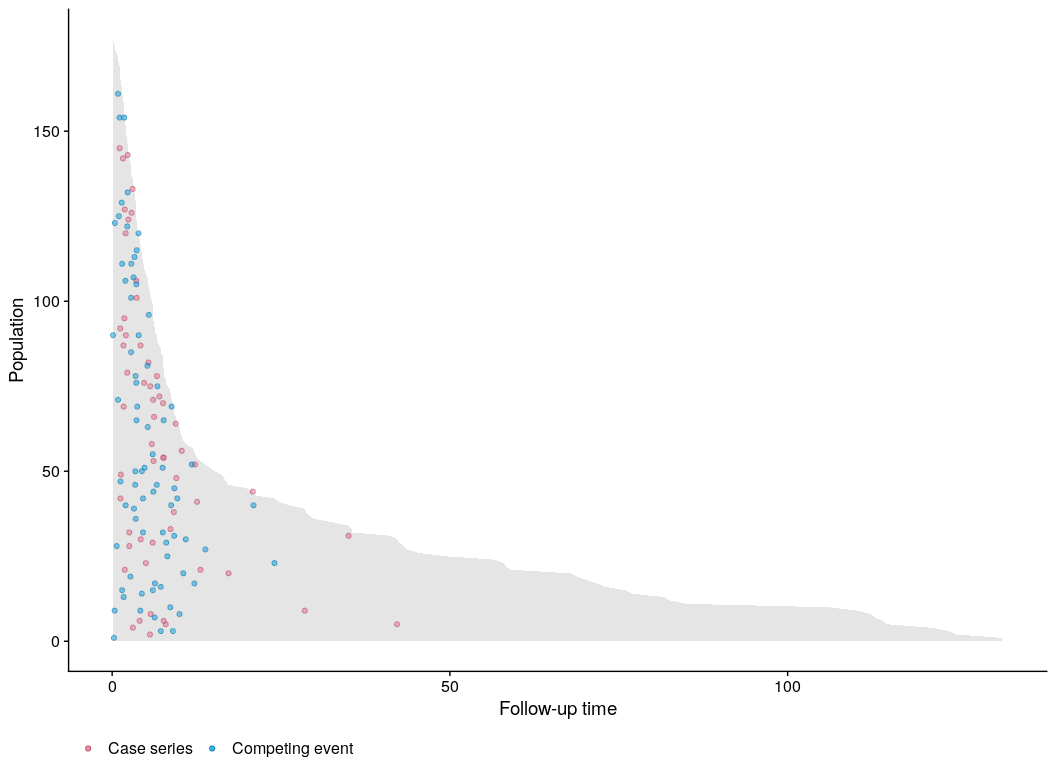## Stratified by Disease

Next we stratify by disease; lymphoblastic or myeloblastic leukemia, abbreviated as ALL and AML, respectively. We must specify the `exposure` variable. Furthermore it is important to properly label the factor variable corresponding to the exposure variable; this will ensure proper labeling of the panels:

``````# create 'popTime' object
popTimeData <- popTime(data = bmtcrr, time = "ftime", exposure = "D")``````
``## 'Status' will be used as the event variable``
``attr(popTimeData, "exposure")``
``##  "D"``
``````plot(popTimeData,
comprisk = TRUE)``````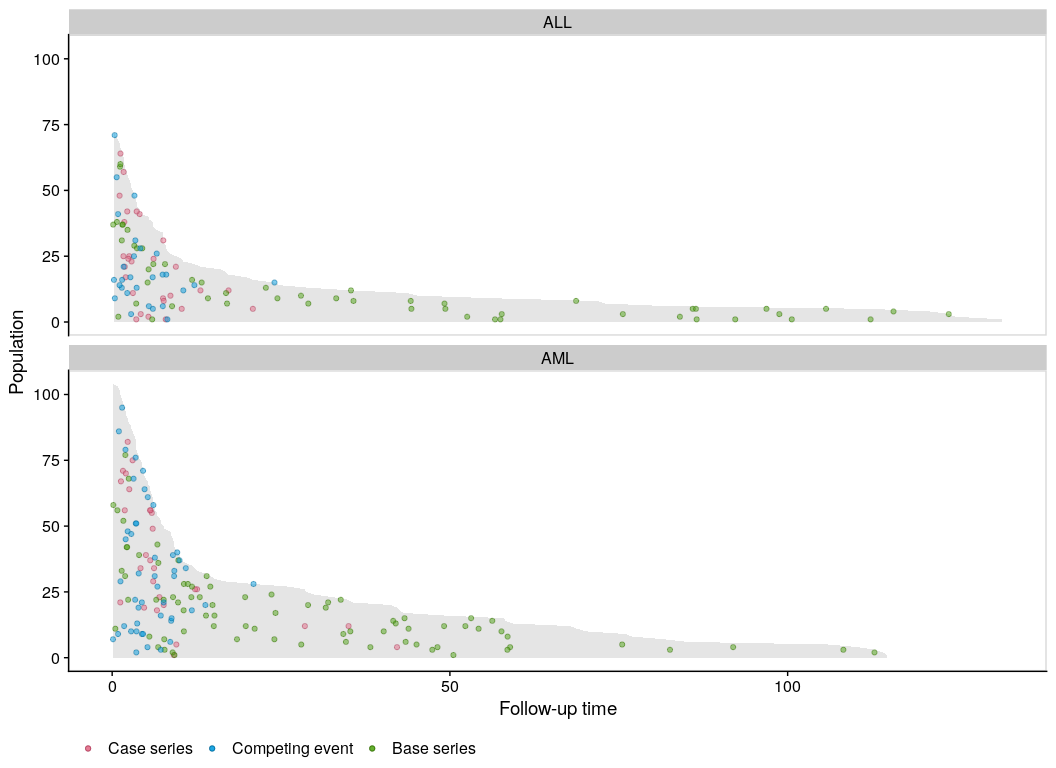## Change color points and legend labels

Here is some code to change color points and legend labels. For a more thorough description, please see the Customizing Population Time Plots vignette.

``````plot(popTimeData,
comprisk = TRUE,
case.params = list(mapping = aes(x = time, y = yc, colour = "Relapse", fill = "Relapse")),
base.params = list(mapping = aes(x = time, y = ycoord, colour = "Base series", fill = "Base series")),
competing.params = list(mapping = aes(x = time, y = yc, colour = "Competing event", fill = "Competing event")),
fill.params = list(name = "Legend Name",
breaks = c("Relapse", "Base series", "Competing event"),
values = c("Relapse" = "blue",
"Competing event" = "red",
"Base series" = "orange"))
)``````
``````## Warning in plot.popTime(popTimeData, add.case.series = TRUE, add.base.series
## = TRUE, : fill.params has been specified by the user but color.params has not.
## Setting color.params to be equal to fill.params.``````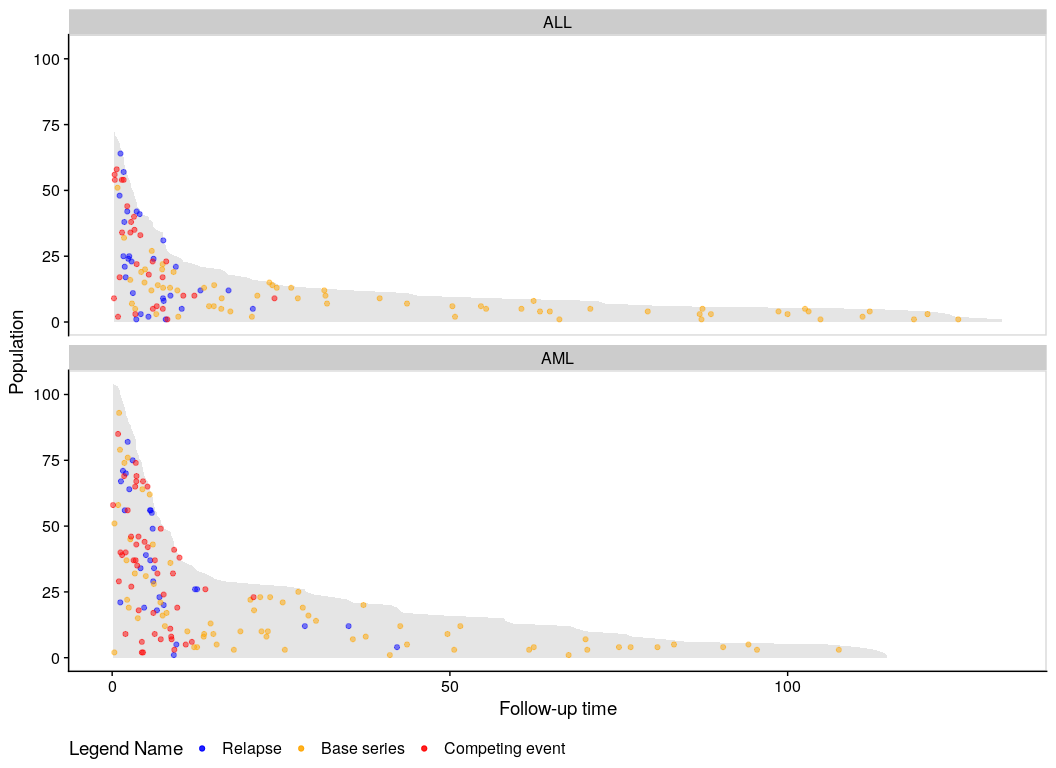# Veteran Data

Below are the steps to create a population time plot for the Veterans’ Administration Lung Cancer study (see `help("veteran", package = "survival")` for more details on this dataset).

``````# veteran data in library(survival)
data("veteran")
str(veteran)``````
``````## 'data.frame':    137 obs. of  8 variables:
##  \$ trt     : num  1 1 1 1 1 1 1 1 1 1 ...
##  \$ celltype: Factor w/ 4 levels "squamous","smallcell",..: 1 1 1 1 1 1 1 1 1 1 ...
##  \$ time    : num  72 411 228 126 118 10 82 110 314 100 ...
##  \$ status  : num  1 1 1 1 1 1 1 1 1 0 ...
##  \$ karno   : num  60 70 60 60 70 20 40 80 50 70 ...
##  \$ diagtime: num  7 5 3 9 11 5 10 29 18 6 ...
##  \$ age     : num  69 64 38 63 65 49 69 68 43 70 ...
##  \$ prior   : num  0 10 0 10 10 0 10 0 0 0 ...``````
``popTimeData <- casebase::popTime(data = veteran)``
``## 'time' will be used as the time variable``
``## 'status' will be used as the event variable``
``class(popTimeData)``
``##  "popTime"    "data.table" "data.frame"``
``plot(popTimeData)``